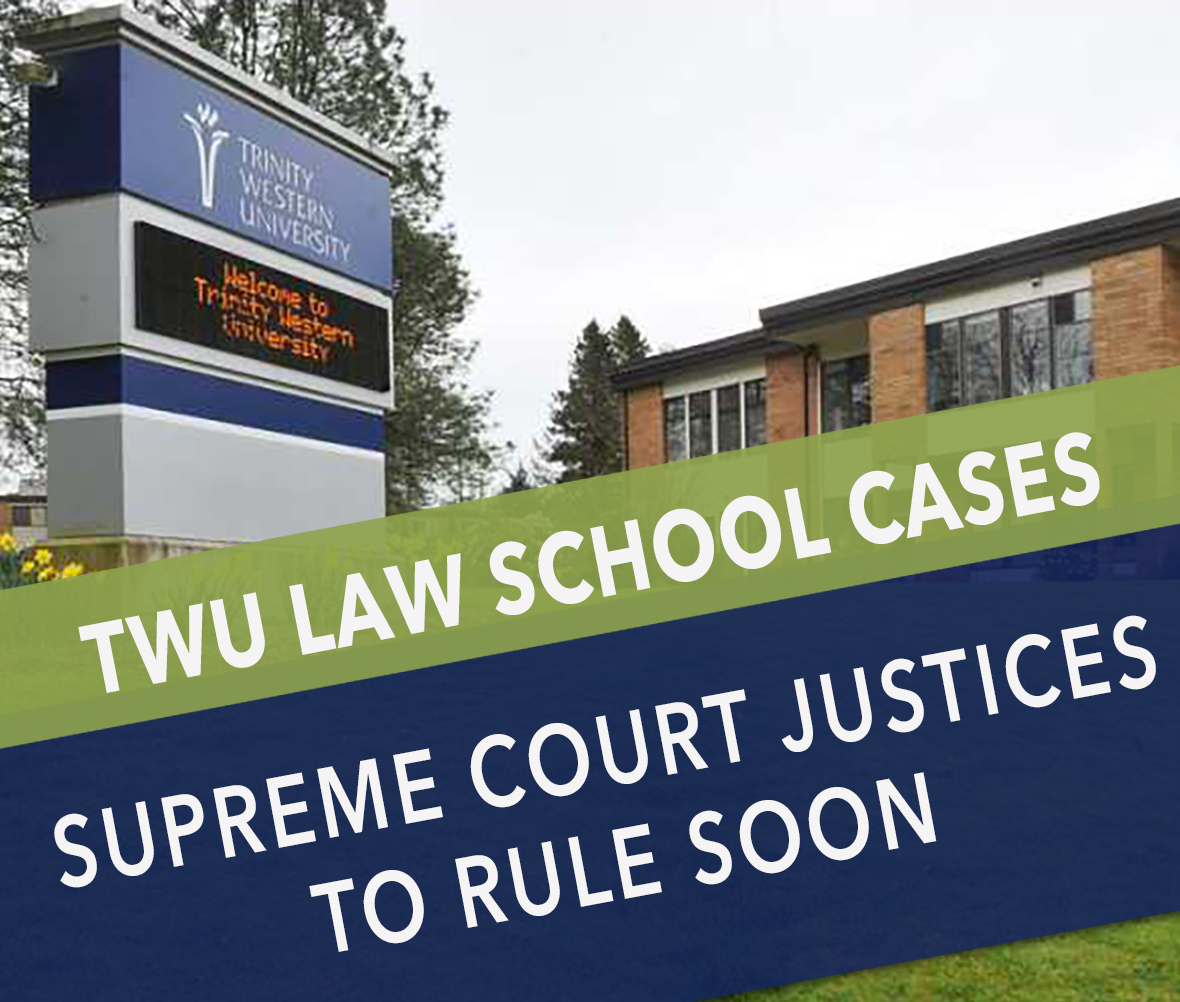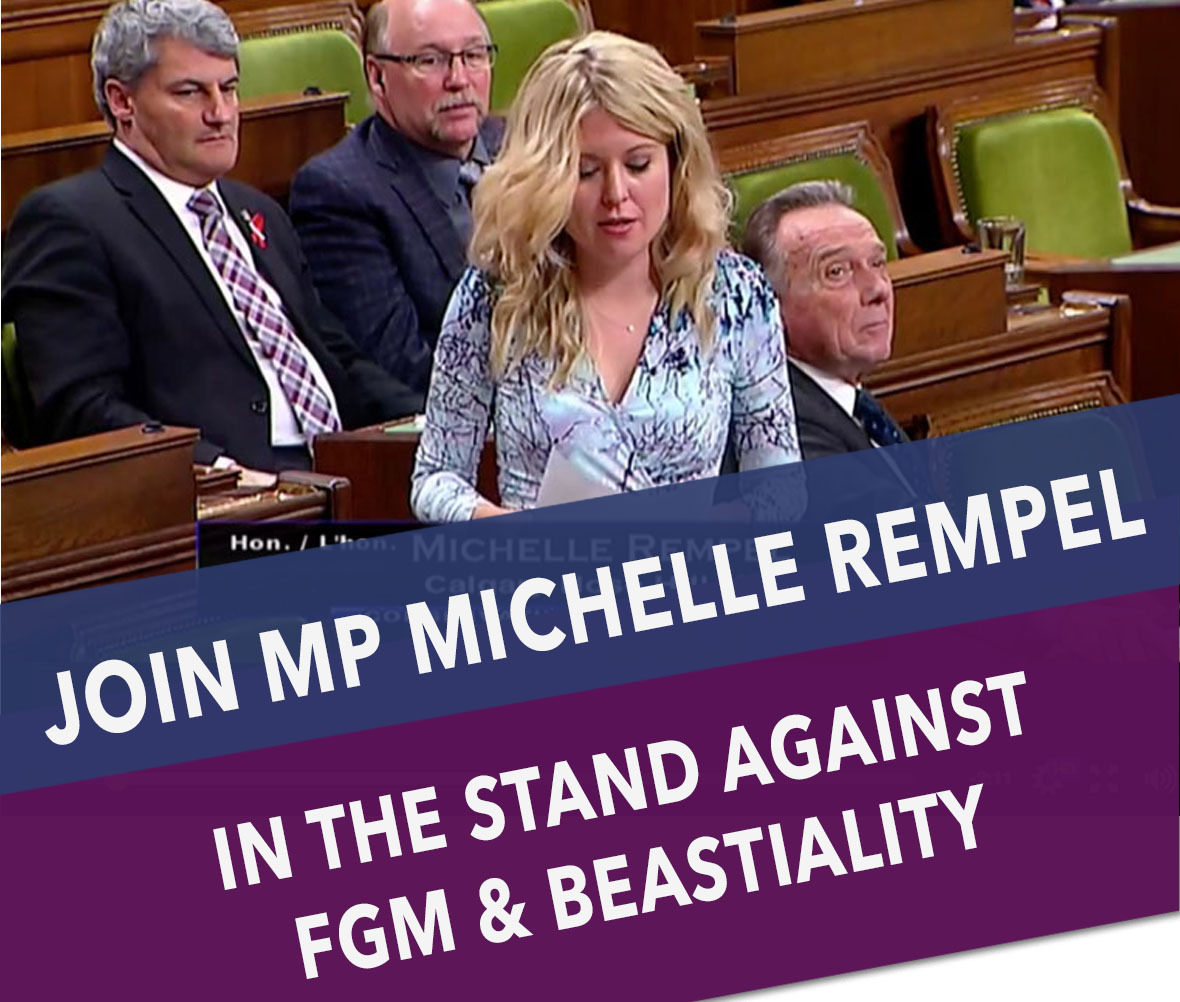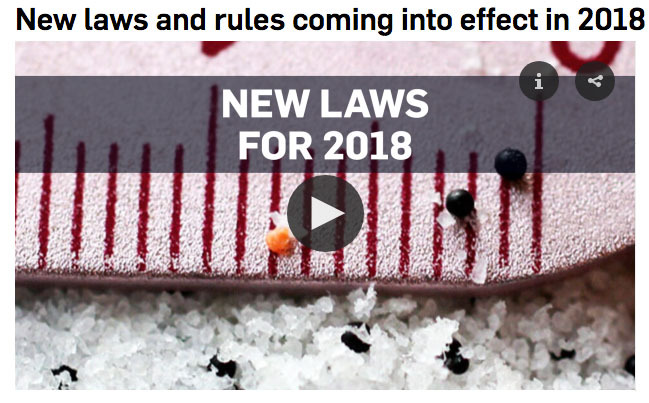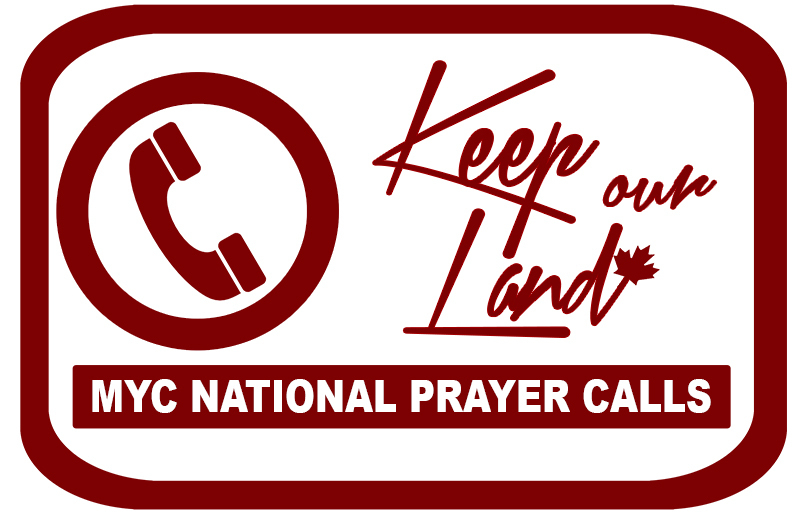Like   Tweet   Pin   +1   in

January 8th, 2018

Hello Canada and bless you as you take on 2018!

As we all hit the ground running this week, fully back at work &/or school, we wanted to share a quick overview of the key things to watch in early 2018.

## MPS ARE HOME UNTIL JAN 29TH

We also wanted to remind you that your Members of Parliament are HOME in their ridings until January 29th. This is good news because it means that you have easier access to them.

During the Christmas/New Years break in the constituency, MPs prioritize meeting with their constituents, aka: YOU :). We encourage you to take advantage of this amazing opportunity.

Below is a brief overview of what you can both be watching and talking to your MP about.## Summer Jobs Program - Opportunity Wed. Jan. 10th

It was announced last month that updates to Canada's Summer Jobs Program for 2018 mean that pro-life and religious organizations may not be able to participate.

Members of Parliament Alex Nuttall and John Brassard are holding a planning meeting on Wednesday, January 10th at 8:30am in Barrie (Bethel Community Church) to take on the issue of funding for the summer jobs program. They need and are asking for support and ideas.

If you are able to attend this significant meeting, please do. Alternatively, please write a letter outlining your concerns about the changes to the program and send it to these two MPs.

MP Alex Nuttall - alex.nuttall@parl.gc.ca

MP John Brassard - john.brassard@parl.gc.ca

 table div table+table+table+table div table{width:100%;padding:0}table div table+table+table+table div table img{width:96.23%;padding:0;float:none}table div table+table+table+table div table td{width:100%;padding:0 1.88% 18px}/* styles */# Bill C-45: The Cannabis Act

 table div table+table+table+table+table+table div table,table.module-5{width:46.04%;float:left;padding:0}table div table+table+table+table+table+table div table a{border:0 none;text-decoration:none}table div table+table+table+table+table+table div table img{width:100%!important;border:0 none;text-decoration:none}table div table+table+table+table+table+table div table td{width:100%;padding:0 20px 20px 0}/* styles */ Before rising for the holidays, Bill C-45 moved to the Senate where it will go through debate, study in a Senate Committee, and voting in the new year. We heard from MPs and Senators alike during our November delegation that the Senate is where this bill might be stopped or held up. Please click here to read our National Update on this to find out how you can contact Senators including e-mail addresses, key information, and a sample letter to use.
 table div table+table+table+table+table+table+table div table{width:100%;padding:0}table div table+table+table+table+table+table+table div table img{width:96.23%;padding:0;float:none}table div table+table+table+table+table+table+table div table td{width:100%;padding:0 1.88% 18px}/* styles */# TWU Law School CasesThe Supreme Court heard the two cases involving Trinity Western University's proposed law school verses the law societies in BC and Ontario on November 30th and December 1st. The Supreme Court's decision on these two cases is expected within a few months time.

Please continue to watch and pray for all involved in this case and that the religious freedom of Canadians would be upheld in the ruling.

 table div table+table+table+table+table+table+table+table+table+table div table{width:100%;padding:0}table div table+table+table+table+table+table+table+table+table+table div table img{width:96.23%;padding:0;float:none}table div table+table+table+table+table+table+table+table+table+table div table td{width:100%;padding:0 1.88% 18px}/* styles */# Help MP Rempel Fight FGM & BeastialityMichelle Rempel is one MP bravely speaking out about some of the most disturbing issues in Canada. She has led the charge to ensure that advisories and warnings about female genital mutilation (FGM) being a crime in Canada remain in Canada's citizenship study guide for new Canadians.

Then, just before the House rose for Christmas break, MP Rempel tabled her private members bill to close the loopholes found in Canada's beastiality laws and ensure that all beastiality acts are covered.

To read more about the fight to keep FGM in the citizenship guide and learn how you can support these efforts, click here.

 table div table+table+table+table+table+table+table+table+table+table+table+table+table div table{width:100%;padding:0}table div table+table+table+table+table+table+table+table+table+table+table+table+table div table img{width:96.23%;padding:0;float:none}table div table+table+table+table+table+table+table+table+table+table+table+table+table div table td{width:100%;padding:0 1.88% 18px}/* styles */# Upcoming Elections, 2018-2019There are a number of elections happening in 2018 that will affect the leadership of our nation at different levels.

Provincial Elections: Ontario will head to the polls for a general election on June 7th, Quebec will do the same on October 1st, and New Brunswick will follow on September 24th. If you live in these provinces, please remember to vote, get involved in your local electoral district, and go out and volunteer for righteous candidates.

Municipal & School Board Trustee Elections: 2018 will see municipal elections in cities and regions all across Canada. Please take some time to look into elections (opportunities) in your area.

2019 Federal Elections: Candidates: Candidates planning to run in the 2019 elections are beginning to prepare their campaigns and we are hoping to see many more godly candidates in the races. This will be a significant opportunity to see a shift in the nation.

 table div table+table+table+table+table+table+table+table+table+table+table+table+table+table+table+table div table{width:100%;padding:0}table div table+table+table+table+table+table+table+table+table+table+table+table+table+table+table+table div table img{width:96.23%;padding:0;float:none}table div table+table+table+table+table+table+table+table+table+table+table+table+table+table+table+table div table td{width:100%;padding:0 1.88% 18px}/* styles */# 2018: New Laws & Rules

For a glimpse at some of the other federal and provincial laws coming in 2019, please click here.

 table div table+table+table+table+table+table+table+table+table+table+table+table+table+table+table+table+table+table div table{width:100%;padding:0}table div table+table+table+table+table+table+table+table+table+table+table+table+table+table+table+table+table+table div table img{width:96.23%;padding:0;float:none}table div table+table+table+table+table+table+table+table+table+table+table+table+table+table+table+table+table+table div table td{width:100%;padding:0 1.88% 18px}/* styles */table div table+table+table+table+table+table+table+table+table+table+table+table+table+table+table+table+table+table+table+table+table div table{width:100%;padding:0}table div table+table+table+table+table+table+table+table+table+table+table+table+table+table+table+table+table+table+table+table+table div table img{width:96.23%;padding:0;float:none}table div table+table+table+table+table+table+table+table+table+table+table+table+table+table+table+table+table+table+table+table+table div table td{width:100%;padding:0 1.88% 18px}/* styles */# GIVING THANKS FOR 2017

In this first e-mail to you of 2018 we wanted to pause and give thanks for all that happened in 2017! Here are the top 10 things we are giving thanks for!

# 10

We saw Bill C-277 - to establish a national palliative care strategy - pass unanimously & receive royal assent.

# 9

We helped support MP Michelle Rempel’s work to see Canada assist the persecuted Yazidi people of Iraq. The Government committed to bringing 1,200 Yazidi refugees in 2017.

# 8

We helped ensure a strong social-conservative voice & vote in the CPC leadership election and celebrated the successful election of a pro-life leader to the role.

# 7

The new Justice Wall website went live to help faciliate hundreds of thousands of intercessors praying for the ending of abortion, human trafficking, the persecuted Church & more.

# 6

MYC took a team of 18 passionate young adults to speak with more than 70 MPs & Senators in sit-down meetings and at our Parliamentary reception.

# 5

MY Canada sent 85+ National Alerts and mobilized Canadians (you!) to many calls to action and prayer to impact the nation.

# 4

Part of our team travelled across Canada to bring MYC’s Get in the Game seminar to over 25 Church communities.

# 3

We began mentoring over 20 amazing Canadians who are planning on running for office in the 2019 and other elections.

# 2

The Church in Canada rose up in defense of freedom of religion and saw section 176 of the Criminal Code preserved.

# 1

Many of our members commemorated Canada’s 150th birthday in prayer through Pray150 (150 days of prayer leading up to July 1st) & at TheCRY Kingston on July 1st. Thank you!

# May God bless you and your loved ones and may God bless Canada!

 table div table+table+table+table+table+table+table+table+table+table+table+table+table+table+table+table+table+table+table+table+table+table+table+table+table div table{width:100%;padding:0}table div table+table+table+table+table+table+table+table+table+table+table+table+table+table+table+table+table+table+table+table+table+table+table+table+table div table img{width:96.23%;padding:0;float:none}table div table+table+table+table+table+table+table+table+table+table+table+table+table+table+table+table+table+table+table+table+table+table+table+table+table div table td{width:100%;padding:0 1.88% 18px}/* styles */table.module-25{width:98.49%;padding:0}table div table+table+table+table+table+table+table+table+table+table+table+table+table+table+table+table+table+table+table+table+table+table+table+table+table+table div table{width:98.49%;float:none;margin-left:auto;margin-right:auto;padding:0}table div table+table+table+table+table+table+table+table+table+table+table+table+table+table+table+table+table+table+table+table+table+table+table+table+table+table div table a{border:0 none;text-decoration:none}table div table+table+table+table+table+table+table+table+table+table+table+table+table+table+table+table+table+table+table+table+table+table+table+table+table+table div table img{width:100%!important;border:0 none;text-decoration:none}table div table+table+table+table+table+table+table+table+table+table+table+table+table+table+table+table+table+table+table+table+table+table+table+table+table+table div table td{width:100%;padding:0}/* styles */

# ANNOUNCEMENTS:

 table div table+table+table+table+table+table+table+table+table+table+table+table+table+table+table+table+table+table+table+table+table+table+table+table+table+table+table+table div table td,table.module-27{width:100%;padding:0}table div table+table+table+table+table+table+table+table+table+table+table+table+table+table+table+table+table+table+table+table+table+table+table+table+table+table+table+table div table{width:100%;float:none;margin-left:auto;margin-right:auto;padding:0}table div table+table+table+table+table+table+table+table+table+table+table+table+table+table+table+table+table+table+table+table+table+table+table+table+table+table+table+table div table a{border:0 none;text-decoration:none}table div table+table+table+table+table+table+table+table+table+table+table+table+table+table+table+table+table+table+table+table+table+table+table+table+table+table+table+table div table img{width:100%!important;border:0 none;text-decoration:none}/* styles */

## First Three Episodes Available On-Line!

The first three episodes of a new national current issues show, with Faytene Grasseschi as host, are now live on YouTube! Click here to go to the YouTube channel. Please consider subscribing. Not only will you get alerts about amazing shows, but you will also be supporting the show. YouTube ranks channels that have more subscribers higher and this means the message the shows carry will travel faster as it gets more followers. Thanks for your support in this way!

FREEDOM: We invite you to watch the first episode featuring Rev. Majed El Shafie by clicking here.

IMMIGRATION: You can watch the second episode on religious freedom by clicking here.

PHYSICIAN CONSCIENCE RIGHTS: The latest episode with guest Dr. Michelle Korvemaker addresses the lack of conscience rights protection for medical professionals. It can be found here.

 table div table+table+table+table+table+table+table+table+table+table+table+table+table+table+table+table+table+table+table+table+table+table+table+table+table+table+table+table+table+table div table{width:100%;padding:0}table div table+table+table+table+table+table+table+table+table+table+table+table+table+table+table+table+table+table+table+table+table+table+table+table+table+table+table+table+table+table div table img{width:96.23%;padding:0;float:none}table div table+table+table+table+table+table+table+table+table+table+table+table+table+table+table+table+table+table+table+table+table+table+table+table+table+table+table+table+table+table div table td{width:100%;padding:0 1.88% 18px}/* styles */## Interested in Running for Election?

We can help! MY Canada is currently conducting a series of trainings and webinars to help godly candidates get started in their campaigns for office. Please e-mail admin@4mycanada.ca if you are interested in learning more.

 table div table+table+table+table+table+table+table+table+table+table+table+table+table+table+table+table+table+table+table+table+table+table+table+table+table+table+table+table+table+table+table+table div table{width:100%;padding:0}table div table+table+table+table+table+table+table+table+table+table+table+table+table+table+table+table+table+table+table+table+table+table+table+table+table+table+table+table+table+table+table+table div table img{width:96.23%;padding:0;float:none}table div table+table+table+table+table+table+table+table+table+table+table+table+table+table+table+table+table+table+table+table+table+table+table+table+table+table+table+table+table+table+table+table div table td{width:100%;padding:0 1.88% 18px}/* styles */## Volunteers Needed:

 table div table+table+table+table+table+table+table+table+table+table+table+table+table+table+table+table+table+table+table+table+table+table+table+table+table+table+table+table+table+table+table+table+table+table div table{width:100%;padding:0}table div table+table+table+table+table+table+table+table+table+table+table+table+table+table+table+table+table+table+table+table+table+table+table+table+table+table+table+table+table+table+table+table+table+table div table img{width:96.23%;padding:0;float:none}table div table+table+table+table+table+table+table+table+table+table+table+table+table+table+table+table+table+table+table+table+table+table+table+table+table+table+table+table+table+table+table+table+table+table div table td{width:100%;padding:0 1.88% 18px}/* styles */table div table+table+table+table+table+table+table+table+table+table+table+table+table+table+table+table+table+table+table+table+table+table+table+table+table+table+table+table+table+table+table+table+table+table+table div table td,table.module-34{width:100%;padding:0}table div table+table+table+table+table+table+table+table+table+table+table+table+table+table+table+table+table+table+table+table+table+table+table+table+table+table+table+table+table+table+table+table+table+table+table div table{width:100%;float:none;margin-left:auto;margin-right:auto;padding:0}table div table+table+table+table+table+table+table+table+table+table+table+table+table+table+table+table+table+table+table+table+table+table+table+table+table+table+table+table+table+table+table+table+table+table+table div table a{border:0 none;text-decoration:none}table div table+table+table+table+table+table+table+table+table+table+table+table+table+table+table+table+table+table+table+table+table+table+table+table+table+table+table+table+table+table+table+table+table+table+table div table img{width:100%!important;border:0 none;text-decoration:none}/* styles */
 /* styles */ The new Justice Wall website is a virtual prayer wall where you can join with others to pray for the ending of abortion, human trafficking and for the persecuted Church around the world. Will you help us build a 24-7 virtual prayer wall for these issues? Please check out the website and share with your friends. Click here or visit www.JusticeWall.com to sign up for your 15-minute prayer slot now.
 table div table+table+table+table+table+table+table+table+table+table+table+table+table+table+table+table+table+table+table+table+table+table+table+table+table+table+table+table+table+table+table+table+table+table+table+table+table div table{width:100%;padding:0}table div table+table+table+table+table+table+table+table+table+table+table+table+table+table+table+table+table+table+table+table+table+table+table+table+table+table+table+table+table+table+table+table+table+table+table+table+table div table img{width:96.23%;padding:0;float:none}table div table+table+table+table+table+table+table+table+table+table+table+table+table+table+table+table+table+table+table+table+table+table+table+table+table+table+table+table+table+table+table+table+table+table+table+table+table div table td{width:100%;padding:0 1.88% 18px}/* styles */table div table+table+table+table+table+table+table+table+table+table+table+table+table+table+table+table+table+table+table+table+table+table+table+table+table+table+table+table+table+table+table+table+table+table+table+table+table+table+table div table{width:100%;padding:0}table div table+table+table+table+table+table+table+table+table+table+table+table+table+table+table+table+table+table+table+table+table+table+table+table+table+table+table+table+table+table+table+table+table+table+table+table+table+table+table div table img{width:96.23%;padding:0;float:none}table div table+table+table+table+table+table+table+table+table+table+table+table+table+table+table+table+table+table+table+table+table+table+table+table+table+table+table+table+table+table+table+table+table+table+table+table+table+table+table div table td{width:100%;padding:0 1.88% 18px}/* styles */MY Canada is hosting national prayer conference calls every second Tuesday at noon EST.

The next call is January 16th, 2018.

 table div table+table+table+table+table+table+table+table+table+table+table+table+table+table+table+table+table+table+table+table+table+table+table+table+table+table+table+table+table+table+table+table+table+table+table+table+table+table+table+table+table div table{width:100%;padding:0}table div table+table+table+table+table+table+table+table+table+table+table+table+table+table+table+table+table+table+table+table+table+table+table+table+table+table+table+table+table+table+table+table+table+table+table+table+table+table+table+table+table div table img{width:96.23%;padding:0;float:none}table div table+table+table+table+table+table+table+table+table+table+table+table+table+table+table+table+table+table+table+table+table+table+table+table+table+table+table+table+table+table+table+table+table+table+table+table+table+table+table+table+table div table td{width:100%;padding:0 1.88% 18px}/* styles */table div table+table+table+table+table+table+table+table+table+table+table+table+table+table+table+table+table+table+table+table+table+table+table+table+table+table+table+table+table+table+table+table+table+table+table+table+table+table+table+table+table+table+table div table{width:100%;padding:0}table div table+table+table+table+table+table+table+table+table+table+table+table+table+table+table+table+table+table+table+table+table+table+table+table+table+table+table+table+table+table+table+table+table+table+table+table+table+table+table+table+table+table+table div table img{width:96.23%;padding:0;float:none}table div table+table+table+table+table+table+table+table+table+table+table+table+table+table+table+table+table+table+table+table+table+table+table+table+table+table+table+table+table+table+table+table+table+table+table+table+table+table+table+table+table+table+table div table td{width:100%;padding:0 1.88% 18px}/* styles */